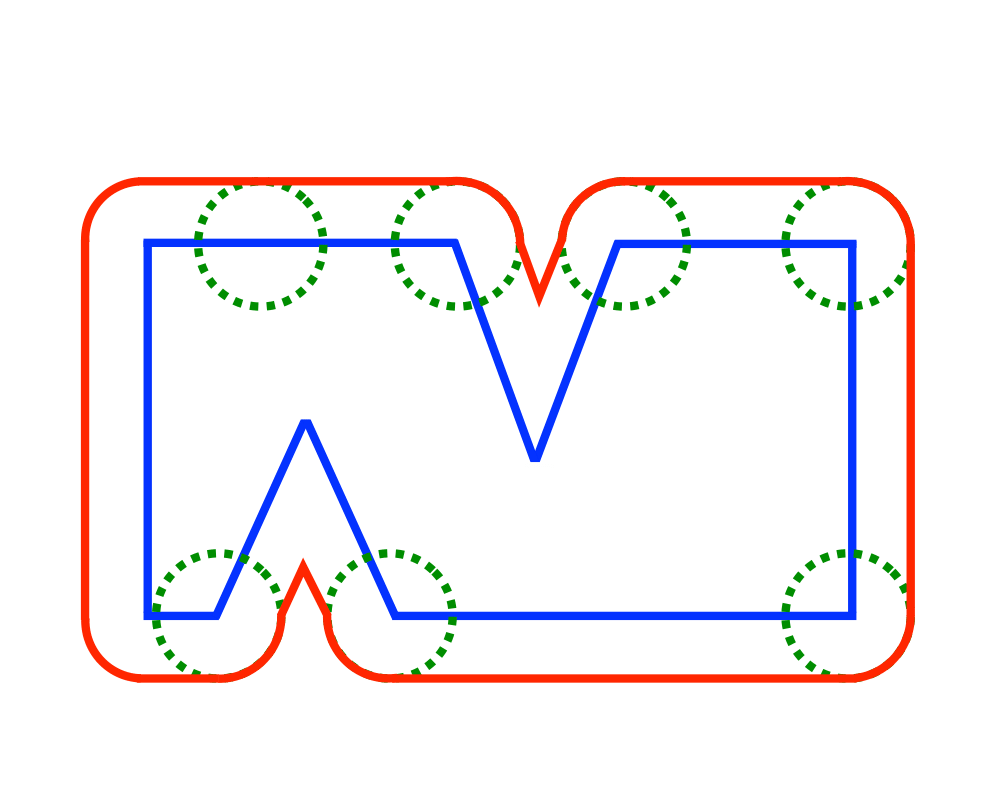# Minkowski SumInputOutput

Input Description: Point sets or polygons $$A$$ and $$B$$, with $$n$$ and $$m$$ vertices, respectively.
Problem: What is the convolution of $$A$$ and $$B$$, ie. the Minkowski sum $$A+B = \{x+y| x\in A, y \in B\}$$?

Excerpt from The Algorithm Design Manual: Minkowski sums are useful geometric operations that can be used to fatten objects in appropriate ways. For example, a popular approach to motion planning for polygonal robots in a room with polygonal obstacles fattens each of the obstacles by taking the Minkowski sum of them with the shape of the robot. This reduces the problem to moving a point from the start to the goal using a standard shortest-path algorithm. Another application is in shape simplification where we fatten the boundary of an object to create a channel and then define as the shape the minimum link path lying within this channel. Similarly, convolving an irregular object with a small circle will help smooth out the boundaries by eliminating minor nicks and cuts.

Go To Main Page